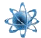xLondon School of Economics
Posts  1 - 1  of  10547102954
How would you answer these questions?
Suppose that the pre-tax demand for and supply of a commodity are governed by the relationships
Qd=300-6p
Qs=80+5p
where Qd = is the quantity demanded. Qs is the quantity supplied and P the unit price of the commodity in GH ¢.
If the government imposes a tax of GH¢ 11 per a unit of the commodity
a. By how much will the price per unit paid by the buyer rise?
b. What will the consumer’s and producer’s share of the tax be?
c. Explain the intuition behind the disparity or otherwise of the distribution of the tax?
d. What will be the total tax revenue harvested by the government as a result of the imposition of the tax?
e. Compute the price elasticity of demand at the pre-tax and post- tax equilibrium level.
f. Compute the price elasticity of supply at the pre-tax and post-tax equilibrium level.
g. Sketch the graph showing both the before and after tax equilibrium level.
Note price is measured in GH¢.